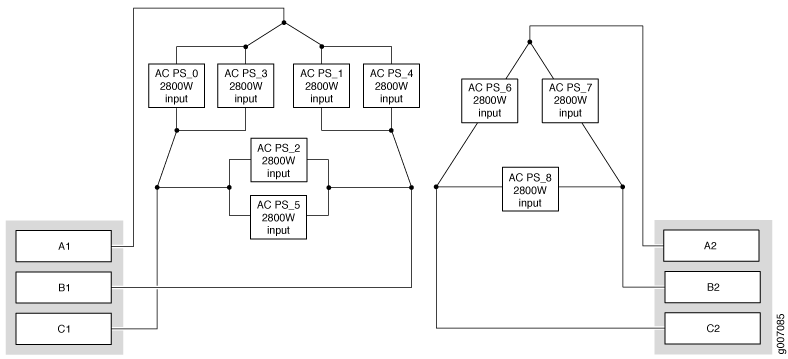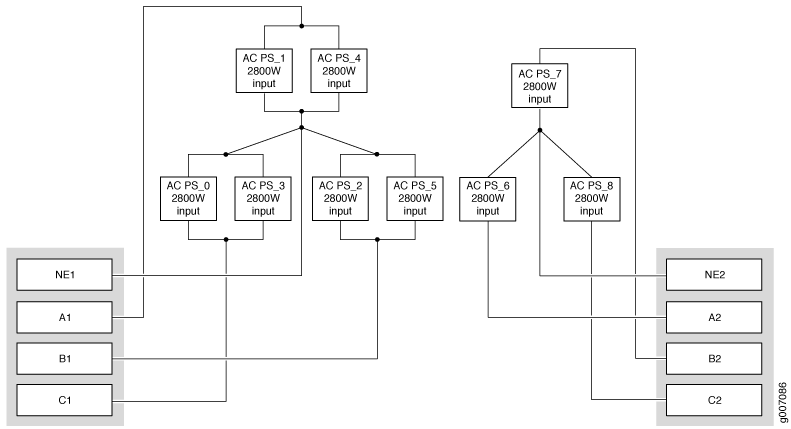Let us know what you think.

Do you have time for a two-minute survey?

# Calculating AC Power Requirements for MX2020 Routers

The information in this topic helps you determine which of the two input ratings for the PSM is suitable for various configurations. You determine suitability by subtracting the total power draw from the maximum output of the PSM. Afterward, you calculate the required input current. Finally, you calculate the thermal output.

We recommend that you provision power according to the maximum input current listed in the power subsystem electrical specifications.

Use the following procedures to calculate the power requirement:

1. Calculate the power requirement.

2. Evaluate the power budget.

3. Calculate input power.

4. Calculate thermal output (BTUs) for cooling requirements.

To calculate the AC power requirements:

1. Calculate the power (usage) using the values, (see MX2020 AC Power Requirements).

2. Evaluate the power budget, including the budget for each configuration if applicable, and check the required power against the maximum output power of available PDM options.

Table 1 lists the three-phase delta and wye feed requirements, maximum input and output power per PSM, and power efficiency.

Table 1: Calculating AC Power Budget

Power Distribution Module

Typical Input Power per PSM

Maximum Input Power per PSM

Maximum Output Power per PSM

Power Supply Module Efficiency

Three-phase delta AC PDM (4 per system)—50 A feed (input #1), and 25 A feed (input #2)

2142 W

2800 W

2500 W

91%

Three-phase wye AC PDM (4 per system)—30 A feed (input #1), and 15 A feed (input #2)

2142 W

2800 W

2500 W

91%

3. To calculate necessary input power for three-phase delta AC PDM, follow the procedure below (see Figure 1).

Figure 1: AC PDM Three-Phase Delta Input PowerAC PSM VIN=200-240 single phase:

1. Two AC PSMs are connected in parallel between two lines.

2. Nominal value of input current for one AC PSM is 2800 W/200 V=14 A.

3. Nominal input current for two AC PSMs is 2*14 A=28 A.

4. Nominal value of line current is 28 A*√3=48.5 A.

5. So, input #1 rated current is 50 A.

6. Only one AC PSM is connected between two lines.

7. Nominal value of input current for one AC PSM is 2800 W/200 V=14 A.

8. Nominal value of line current is 14 A*√3=24.5 A.

9. Current rating for input #2 is 25 A.

4. To calculate necessary input power for three-phase wye AC PDM, follow the procedure below (see Figure 2).

Figure 2: AC PDM Three-Phase Wye Input PowerAC PSM VIN=200-240 single phase:

1. Two AC PSMs are connected in parallel between two lines and neutral.

2. Nominal value of input current for one AC PSM is 2800 W/200 V=14 A.

3. Nominal input current for two AC PSMs is 2*14 A=28 A.

4. Nominal value of line current is 28 A.

5. So, input #1 rated current is 28 A.

6. Only one AC PSM is connected between two lines and neutral.

7. Nominal value of input current for one AC PSM is 2800 W/200 V=14 A.

8. Nominal value of line current is 14 A.

9. Current rating for input #2 is 14 A.

5. Calculate thermal output (BTUs). Multiply the input power requirement (in watts) by 3.41 as shown in Table 2.

Table 2: Calculating AC Thermal Output

Power Distribution Module

Thermal Output (BTUs per hour)

MX2020 Three-phase delta AC PDM

Maximum power divided by 0.91 * 3.41 = BTU/hr.

Input power = Maximum power divided by 0.91

Refer to MX2020 AC Power Requirements to calculate maximum power, which is dependent on configuration and temperature.

MX2020 Three-phase wye AC PDM

Maximum power divided by 0.91 * 3.41 = BTU/hr.

Input power = Maximum power divided by 0.91

Refer to MX2020 AC Power Requirements to calculate maximum power, which is dependent on configuration and temperature.